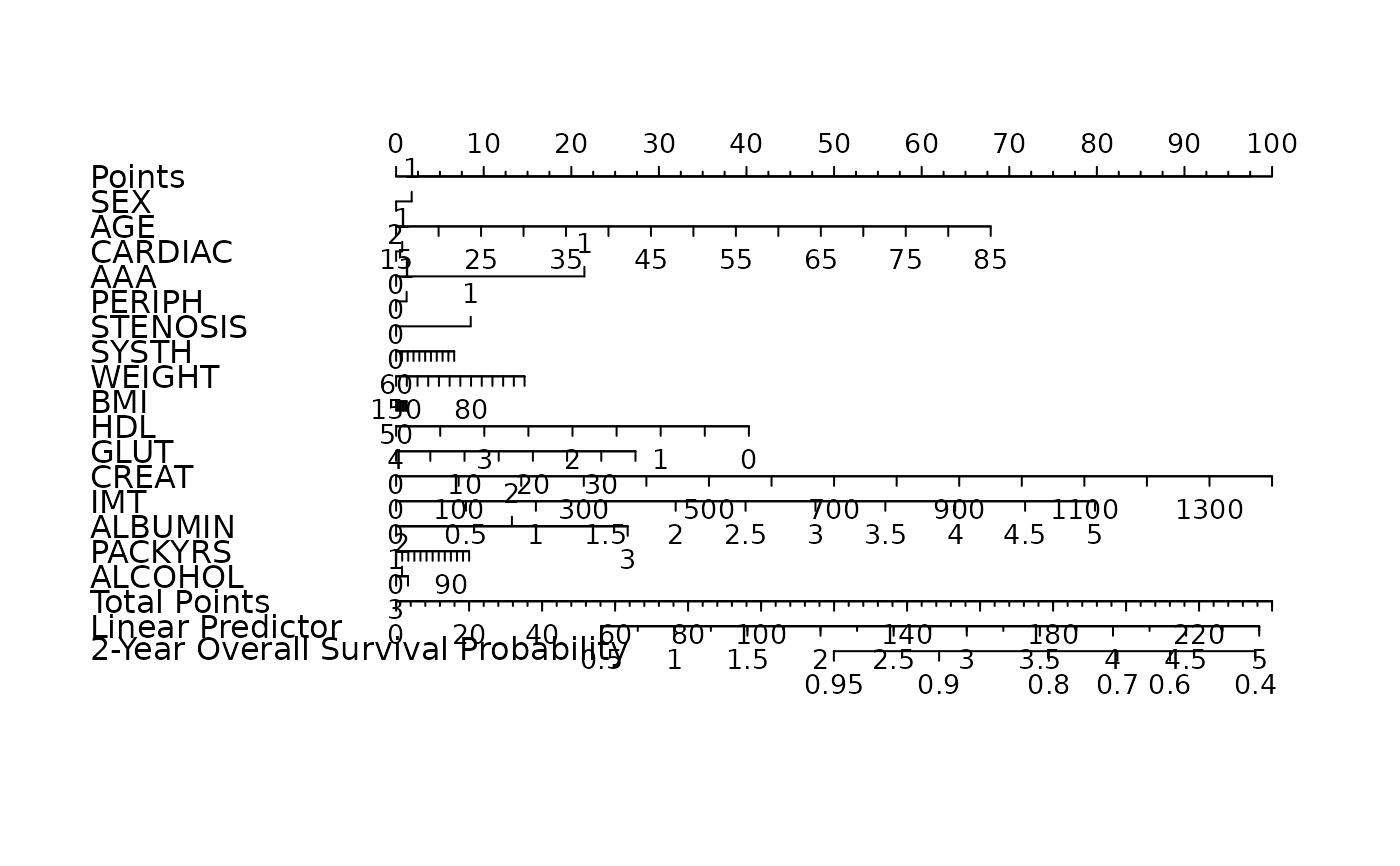Automatic model selection for high-dimensional Cox models with lasso penalty, evaluated by penalized partial-likelihood.

fit_lasso(x, y, nfolds = 5L, rule = c("lambda.min", "lambda.1se"),
seed = 1001)

## Arguments

x Data matrix. Response matrix made by Surv. Fold numbers of cross-validation. Model selection criterion, "lambda.min" or "lambda.1se". See cv.glmnet for details. A random seed for cross-validation fold division.

## Examples

data("smart")
x <- as.matrix(smart[, -c(1, 2)])
time <- smart$TEVENT event <- smart$EVENT
y <- survival::Surv(time, event)

fit <- fit_lasso(x, y, nfolds = 5, rule = "lambda.1se", seed = 11)

nom <- as_nomogram(
fit, x, time, event,
pred.at = 365 * 2,
funlabel = "2-Year Overall Survival Probability"
)

plot(nom)